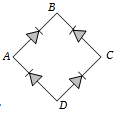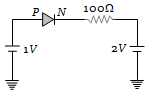Which is the wrong statement in following sentences?

A device in which P and N-type semiconductors are used is more useful then a vacuum type because

(a) Power is not necessary to heat the filament

(b) It is more stable

(c) Very less heat is produced in it

(d) Its efficiency is high due to a high voltage across the junction

Concept Questions :-

Applications of PN junction

Difficulty Level:

In the diagram, the input is across the terminals A and C and the output is across the terminals B and D, then the output is(a) Zero
(b) Same as input
(c) Full wave rectifier
(d) Half wave rectifier

Concept Questions :-

Rectifier
High Yielding Test Series + Question Bank - NEET 2020

Difficulty Level:

The current through an ideal PN-junction shown in the following circuit diagram will be(a) Zero
(b) 1 mA
(c) 10 mA
(d) 30 mA

Concept Questions :-

PN junction
High Yielding Test Series + Question Bank - NEET 2020

Difficulty Level:

If a full wave rectifier circuit is operating from 50 Hz mains, the fundamental frequency in the ripple will be

(a) 50 Hz                           (b) 70.7 Hz
(c) 100 Hz                         (d) 25 Hz

Concept Questions :-

Rectifier
High Yielding Test Series + Question Bank - NEET 2020

Difficulty Level:

A diode having potential difference 0.5 V across its junction which does not depend on current, is connected in series with resistance of 20 $\mathrm{\Omega }$ across source. If 0.1 A passes through resistance then what is the voltage of the source

(a) 1.5 V                     (b) 2.0 V
(c) 2.5 V                     (d) 5 V

Concept Questions :-

PN junction
High Yielding Test Series + Question Bank - NEET 2020

Difficulty Level:

When NPN transistor is used as an amplifier

(a) Electrons move from base to collector

(b) Holes move from emitter to base

(c) Electrons move from collector to base

(d) Holes move from base to emitter

Concept Questions :-

Applications of Transistor
High Yielding Test Series + Question Bank - NEET 2020

Difficulty Level:

The phase difference between input and output voltages of a CE circuit is

(a) 0$°$                          (b) 90$°$
(c) 180$°$                       (d) 270$°$

Concept Questions :-

Applications of Transistor
High Yielding Test Series + Question Bank - NEET 2020

Difficulty Level:

The emitter-base junction of a transistor is …… biased while the collector-base junction is ……. biased

(a) Reverse, forward                        (b) Reverse, reverse
(c) Forward, forward                        (d) Forward, reverse

Concept Questions :-

Transistor
High Yielding Test Series + Question Bank - NEET 2020

Difficulty Level:

In an NPN transistor the collector current is 24 mA. If 80% of electrons reach collector its base current in mA is

1. 36                       2. 26
3. 16                       4. 6

Concept Questions :-

Transistor
High Yielding Test Series + Question Bank - NEET 2020

Difficulty Level:

If $\mathrm{\alpha }$ = 0.98 and current through emitter ${\mathrm{i}}_{\mathrm{e}}$ = 20 mA, the value of $\mathrm{\beta }$ is
(a) 4.9                  (b) 49
(c) 96                   (d) 9.6

Concept Questions :-

Transistor